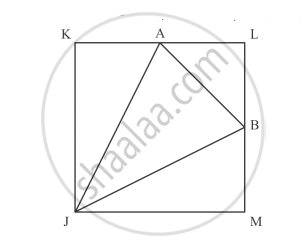# In the Fig. Below, Jklm is a Square with Sides of Length 6 Units. Points a and B Are the Mid- Points of Sides Kl and Lm Respectively. If a Point is Selected at Random from the Interior of the Square. What is the Probability that the Point Will Be Chosen from the Interior of δJab? - Mathematics

In the Fig. below, JKLM is a square with sides of length 6 units. Points A and B are the
mid- points of sides KL and LM respectively. If a point is selected at random from the
interior of the square. What is the probability that the point will be chosen from the interior of ΔJAB?#### Solution

Given: JKLM is a square with sides of length 6units. Points A and B are the midpoints of sides KL and ML respectively. If a point is selected at random from the interior of the square

To find: Probability that the point will be chosen from the interior of ΔJAB.

We the following figureArea of square JLKM is equal to

= 6^2

= 36 sq units

Now we have

area(triangleKAJ)= 1/2xxAKxxKJ

=1/2xx3xx6

=9 units2

area(triangleJMB)=1/2xxJMxxBM

=1/2xx6xx3

=9/2 units2

Now area of the triangle AJB

area(triangleAJB) = 36-9-9-9/2

=27/2units2

We know that Probability

="Number of favourable event"/"Total number of event"

= (27/2)/36

= 27/(2xx36)

= 3/8

Hence the Probability that the point will be chosen from the interior of ΔAJB is 3/8.

Concept: Basic Ideas of Probability
Is there an error in this question or solution?

#### APPEARS IN

RD Sharma Class 10 Maths
Chapter 16 Probability
Exercise 16.2 | Q 5 | Page 33